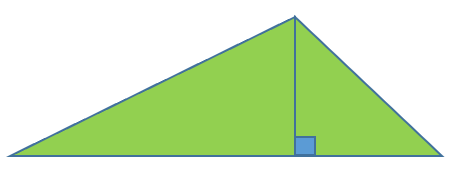# Area of a Triangle Using Trigonometry Worksheets

How to Find the Area of Triangle by Using Trigonometry - We all know the general formula for calculating the area of a triangle. It is expressed as: A= 1/2 b x h. In the above formula, 'b' is defined as the base or length of a side, where 'h' is defined as the height draw to the side. Reading from the above formula, we say that the area of the triangle is half the length of one side times the height drawn with it.However, there is another way to measure the area of a triangle. Trigonometry is not only used to find different angles and sides of a right-angled triangle; we use trigonometry to calculate the area of a triangle. Using the above right-angled triangle, we can say, sin C = h/b. Multiplying the above equation by b, we get:b sin C = h. The height of the triangle can now be written as b sin C. Replacing this new value of height in the general formula for calculating the area of a triangle. A= 1/2 ab sin C. Where the letters 'a' and 'b' express any two sides of the triangle, and C represents the included angle. With this derived formula, we aren't required to find the height of the triangle. If you know the two sides and their included angle, you can substitute these values in this formula to find the area of the triangle.

• ### Basic Lesson

Guides students solving equations that involve an Area of a Triangle Using Trig. Demonstrates answer checking. Given the triangle at the right, find its area. Express the area rounded to three decimal places.

• ### Intermediate Lesson

Demonstrates how to solve more difficult problems. Given the triangle at the right BC = 12, AC = 10 and ∠C84 find its area. Express the area rounded to three decimal places.

• ### Independent Practice 1

A really great activity for allowing students to understand the concept we are exploring here. Example: In an isosceles ⃤ , the two equal sides each measure 46 meters, and they include an angle of 4630°. Find the area of the isosceles triangle, to the nearest sq. meter.

• ### Independent Practice 2

Students find the Area of a Triangle Using Trig.s in assorted problems. The answers can be found below.

• ### Homework Worksheet

Students are provided with problems to achieve the concepts that come with these skills.

• ### Skill Quiz

This tests the students ability to evaluate the area of triangles.